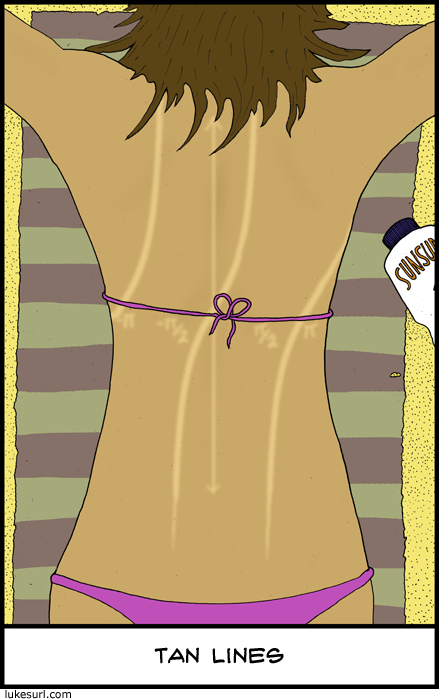Search IntMath
Close

# Tan lines

By Murray Bourne, 28 Nov 2010

This is fun. And the best part - it's accurate!

If you don't get it, see Graphs of tan, cot, sec and csc.Image source: LukeSurl.com

He's got some other math-flavored comics (search "math").

See the 1 Comment below.

### Comment Preview

HTML: You can use simple tags like <b>, <a href="...">, etc.

To enter math, you can can either:

1. Use simple calculator-like input in the following format (surround your math in backticks, or qq on tablet or phone):
a^2 = sqrt(b^2 + c^2)
(See more on ASCIIMath syntax); or
2. Use simple LaTeX in the following format. Surround your math with $$ and $$.
$$\int g dx = \sqrt{\frac{a}{b}}$$
(This is standard simple LaTeX.)

NOTE: You can mix both types of math entry in your comment.